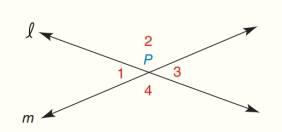Chapter 1.CT, Problem 15CT### Elementary Geometry for College St...

6th Edition
Daniel C. Alexander + 1 other
ISBN: 9781285195698

#### Solutions

Chapter
Section### Elementary Geometry for College St...

6th Edition
Daniel C. Alexander + 1 other
ISBN: 9781285195698
Textbook Problem
1 views

# If m ∠ 1 = 2 x − 3   and   m ∠ 3 = 3 x − 28 , find: a) x _______ b) m ∠ 1 _______To determine

To find:

The values of x and m1.

Explanation

Given:

The given figure is,

m1=2x3andm3=3x28

Calculation:

Consider the given figure,

Figure (1)

From the figure, 1and3 are forming linear pair

To determine

To find:

The values of m1.

### Still sussing out bartleby?

Check out a sample textbook solution.

See a sample solution

#### The Solution to Your Study Problems

Bartleby provides explanations to thousands of textbook problems written by our experts, many with advanced degrees!

Get Started

#### Let g be the function defined by g(u) = (3u 2)3/2. Find g(1), g(6), g(113), and g(u + 1).

Applied Calculus for the Managerial, Life, and Social Sciences: A Brief Approach

#### Evaluate the integral. 01(18v3+16v7)dv

Single Variable Calculus: Early Transcendentals

#### Given that for all x, a power series for is:

Study Guide for Stewart's Multivariable Calculus, 8th

#### Define fraud and explain the safeguards that exist to prevent it.

Research Methods for the Behavioral Sciences (MindTap Course List)